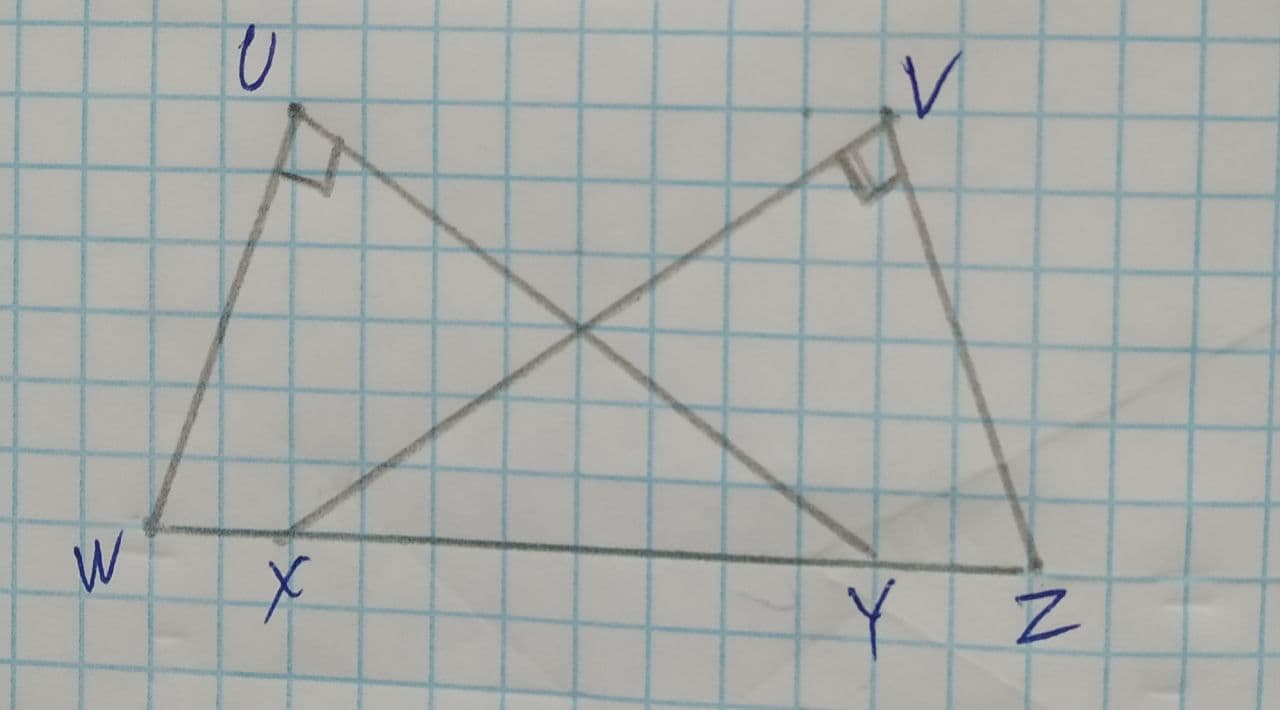Question# \overline{WU}\cong\overline{ZV}\ and\ \overline{WX} = \overline{YZ}. \angle U, \angle V are right angles, find congruence criteria used to prove that the triangles are congruent.

Congruence
ANSWEREDTo find: congruent criteria used to prove that the triangles are congruent.
Given information: $$\displaystyle\overline{{{W}{U}}}\stackrel{\sim}{=}\overline{{{Z}{V}}}\ {\quad\text{and}\quad}\ \overline{{{W}{X}}}=\overline{{{Y}{Z}}}$$. $$\displaystyle\angle{U},\angle{V}$$ are right angles2021-07-31
Consider $$\displaystyle\triangle{W}{U}{Y},\triangle{Z}{V}{X}$$
$$\displaystyle\overline{{{W}{U}}}\stackrel{\sim}{=}\overline{{{V}{Z}}}$$ (Given)
As $$\displaystyle\angle{U},\angle{V}$$ are right angles,
$$\displaystyle\angle{U}\stackrel{\sim}{=}\angle{V}$$ (right angles are congruent)
$$\displaystyle\overline{{{W}{X}}}\stackrel{\sim}{=}\overline{{{Y}{Z}}}$$ (Given)
Add $$\displaystyle\overline{{{X}{Y}}}$$ to both sides.
$$\displaystyle\overline{{{W}{X}}}+\overline{{{X}{Y}}}=\overline{{{Y}{Z}}}+\overline{{{X}{Y}}}$$
$$\displaystyle\Rightarrow\overline{{{W}{Y}}}=\overline{{{X}{Z}}}$$
So,
$$\displaystyle\overline{{{W}{Y}}}\stackrel{\sim}{=}\overline{{{X}{Z}}}$$ (line segments of equal measures are congruent)
Therefore,
$$\displaystyle\triangle{W}{U}{Y},\triangle{Z}{V}{X}$$ (RHS congruence criteria)
Also,
Congruent triangles basically overlap each other.
So, $$\displaystyle\triangle{W}{U}{Y},\triangle{Z}{V}{X}$$ is a pair of overlapping triangles.